# Optimizing for a Target Spatial Distribution

### Introduction

This knowledge base article will demonstrate how to use FRED Optimum’s optimization capability to modify a system and achieve a desired irradiance distribution. The system being modified is a PMMA light pipe. Six variables control the shape of the light pipe and the optimization merit function aberration is the RMS difference between the current light distribution and the ideal light distribution. The optimization merit function aberration is a user-defined script.

This example uses a source to define the target irradiance - an extension of this article explains how to use a bitmap to define a target and is available here.

### System Layout

The rear and profile views of the light pipe geometry are shown in the figure below.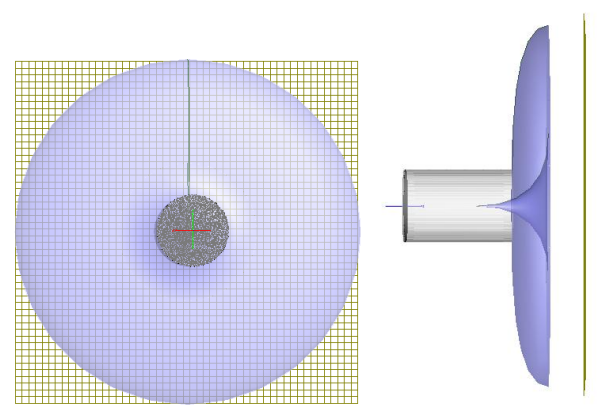As shown below, two surfaces in the light pipe are surfaces of revolution formed from two 2nd degree NURB curves. The optimization process uses the positions and weights of some of the control points as variables in the optimization process to deform the shapes of the light pipe surfaces. Shown schematically, the green dots are the control points that will be manipulated during the optimization.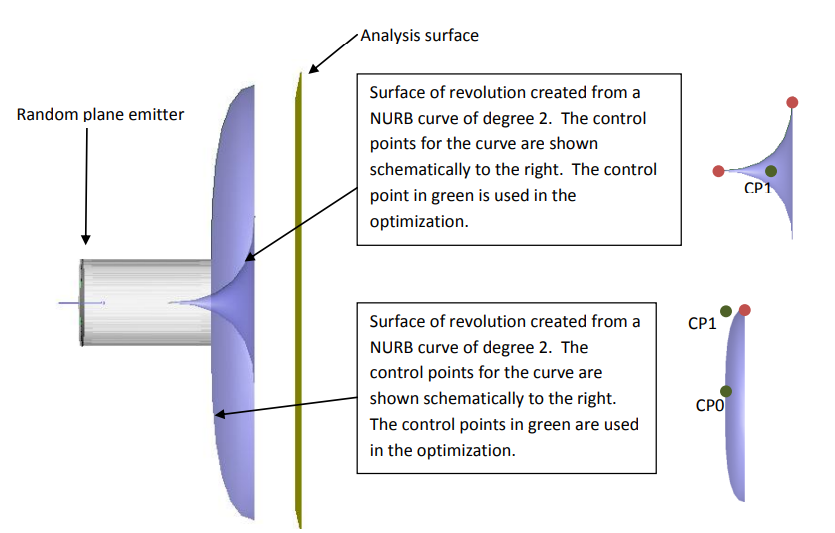A random plane emitter is embedded in the cylindrical tube portion of the light pipe. In the starting configuration, the irradiance distribution at the analysis surface is shown below.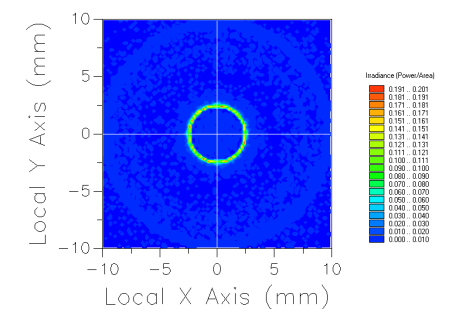The desired distribution to be achieved after optimization is shown below.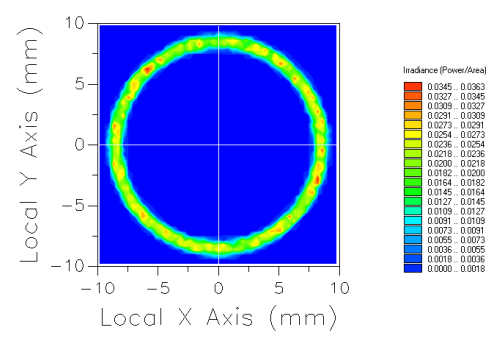Notice in the FRED file that there is a second source called IdealDistribution with an associated analysis plane called IdealAnalysis. In the FRED model, this source represents the ideal distribution to be achieved at the end of the optimization.

### Optimization Variables

As mentioned previously, the variables in the optimization will be the positions and weights of several control points in the base curves defining the surfaces of revolution in the light pipe. The variables are listed below and can be accessed within the FRED file itself by navigating to Menu > Optimize > Define/Edit.

 Variable Lower Limit Upper Limit Fraction Step Geometry.Shell.Curve1 CP0 Z-Position -4 1 0.5 CP1 Y-Position 0 10 0.5 CP1 Weight 0 2 0.5 Geometry.TIRSurf.Curve 2 CP1 Y-Position 0 4 0.5 CP1 Z-Position -4 0 0.5 CP1 Weight 0 2 0.5 CP2 Z-Position -4 0 0.5

### The merit function aberration being used is a User-defined script, since there currently exists no default method for importing a target distribution into the optimizer. The script used to implement the aberration to target a distribution is provided below with comments.

```<span style="font-weight: normal;"></span>Sub EvalMzrAber( ByVal g_ana&, ByRef g_aber#, ByRef g_success As Boolean )

Dim arnStats As T_ARN_2D_CELL_STATS
Dim anaIdeal As Long
Dim rndArn As Long, idealArn As Long, count As Long, ii As Long, jj As Long
Dim data() As Double, totDiffPower As Double, totRndPower As Double, totIdealPower As Double
Dim dBdirX As Double, dBdirY As Double, dBdirZ As Double
Dim success As Boolean

'find node number of the ideal source analysis plane
anaIdeal = FindFullName( "Analysis Surface(s).IdealAnalysis" )

'store the ideal irradiance distribution in an ARN and normalize the power
count = IrradianceToARN( anaIdeal, "idealARN", idealArn )
totIdealPower = ARNComputeTotalPower( idealArn )
ARNGetDataAsDoubleArray idealArn, data()
For ii = 0 To UBound(data,1)
For jj = 0 To UBound(data,2)
data(ii,jj) = data(ii,jj)/totIdealPower
Next jj
Next ii
ARNSetDataAsDoubleArray idealArn, data()

'store the traced irradiance distribution in an ARN and renormalize the power
count = IrradianceToARN( g_ana, "randomARN", rndArn )
totRndPower = ARNComputeTotalPower( rndArn )
ARNGetDataAsDoubleArray rndArn, data()
For ii = 0 To UBound(data,1)
For jj = 0 To UBound(data,2)
data(ii,jj) = data(ii,jj)/totRndPower
Next jj
Next ii
ARNSetDataAsDoubleArray rndArn, data()

'difference the two ARN. Results are stored in idealArn node.
ARNLinearCombination( -1, idealArn, 1, rndArn )
ARNGetDataAsDoubleArray idealArn, data()
For ii = 0 To UBound(data,1)
For jj = 0 To UBound(data,2)
data(ii,jj) = Abs( data(ii,jj) )
Next jj
Next ii
ARNSetDataAsDoubleArray idealArn, data()

'get statistics and set aberration value
ARNCompute2DCellStatistics idealArn, arnStats
g_aber = Sqr(arnStats.AvgSqNonZeroCellVal)

'delete the ARN nodes (memory management)
success = ARNDelete( idealArn )
success = ARNDelete( rndArn )

g_success = totRndPower > 0

End Sub
```

### Optimization Results

The comparison between the light pipe exit distribution and the target distribution are shown below for three optimization sets of 20 iterations each using all variables.  The top image is the resulting distribution for the lightpipe after optimization and the bottom distribution is the ideal result that was being targeted.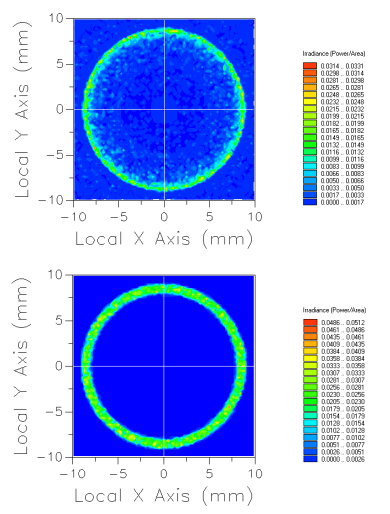Example FRED File: targetedDistributionOptimization.frd